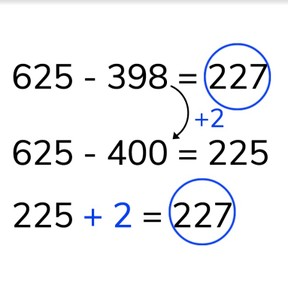Subtraction to 1,000 via compensation method

# Subtraction to 1,000 via compensation method8,000 schools use Gynzy92,000 teachers use Gynzy1,600,000 students use Gynzy

## General

Students learn, when subtracting numbers to 1,000 to use the compensation method, whereby they round one of the numbers to the nearest tens or hundreds number and then to add what they rounded to the difference.

2.NBT.B.7

## Relevance

It is important to be able to do this so that students are able to easily calculate more difficult subtraction problems.

## Introduction

Explain that hundreds are a group of one hundred and have zeros in the tens and ones places- its called a round number. Ask students if they can name any hundreds numbers. Practice rounding numbers to the nearest hundreds number. The interactive whiteboard shows some melons with random numbers. Ask students if they need to round them up or down. 94 becomes 100, 195 becomes 200, and so on.

## Development

Explain to students how to use the compensation method on subtraction problems to 1,000. A problem like 625 - 398 can be solved easily when you compensate the numbers. Select the number that is closest to a hundreds number and round it to the nearest hundred. The number 398 is rounded to 400 by adding 2 more. The rounded subtraction problem becomes 625 -400. The two you added to 398 are now added to the difference, so 225 + 2 = 227. The difference is the same. Explain to students that sometimes you round the first number, and sometimes you round the second number in the subtraction problem- they must look carefully to see which number is closest to a hundreds number. Ask students to do a selection of subtraction problems. Then explain how you can also round to a tens number. With the subtraction problem 400 - 39 you round the second number to the nearest tens number which is 40. The next steps are exactly the same as with a hundreds number. Ask students to solve a few subtraction problems in which they must round a tens number. Check that students are able to determine which rounded subtraction problem matches a given subtraction problem. You can erase the grey boxes to check their answers. Using the number line, show that you must always look carefully to see which number to round.

Check that students are able to subtract to 1,000 via the compensation method by asking the following questions:
- Which ways of rounding can you use to solve subtraction problems to 1,000? (rounding to tens or hundreds)
- Round the following numbers to their nearest hundreds number: 399 : 596 : 92 : 798 : 495
- How can you solve 327 - 95?
- What is the most important thing to remember when subtracting via compensation? (what you rounded up or down, needs to be added back)

## Guided practice

Students are first asked to solve by first rounding to a hundreds or tens number and to select the correct answer. Next they are asked to round numbers to to a nearest hundreds number and then to fill in the difference.

## Closing

Ask students to determine which rounded subtraction problem matches the given subtraction problems and to drag them to the blue boxes. Ask why they think they match together. Discuss why it is useful to round a subtraction number. Remind students that what they add to a number they must take away, or what they take away must be taken away as well.

## Teaching tips

Students who have difficulty with this learning goal can use MAB materials or other manipulatives to make rounding more visible. That helps to make the action of rounding more concrete and shows students that what they round must be accounted for.

## Instruction materials

Optional: MAB blocks or other manipulatives

### The online teaching platform for interactive whiteboards and displays in schools

• Save time building lessons

• Manage the classroom more efficiently

• Increase student engagement